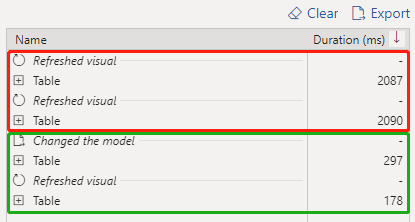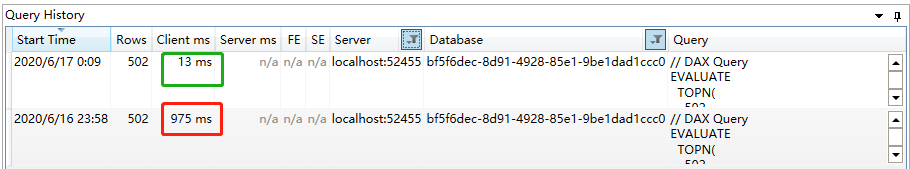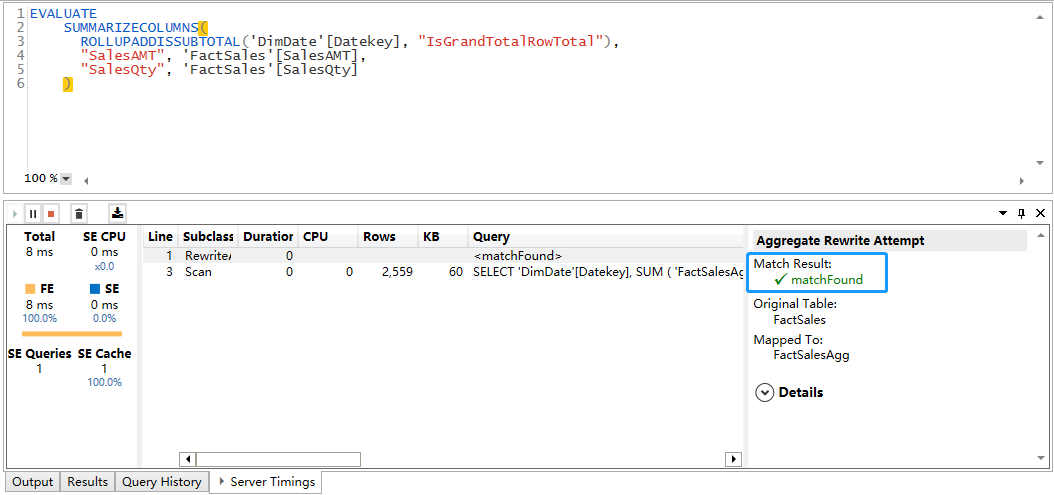# Power BI 的大数据处理方案：聚合

### 前奏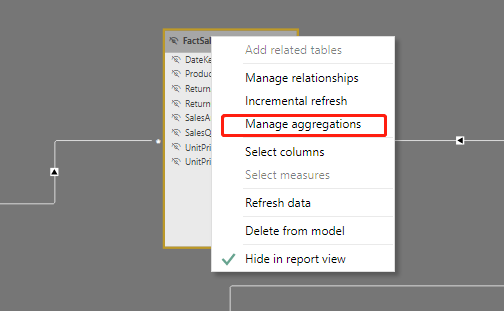（图：Power BI Desktop中的”管理聚合”)

### 原理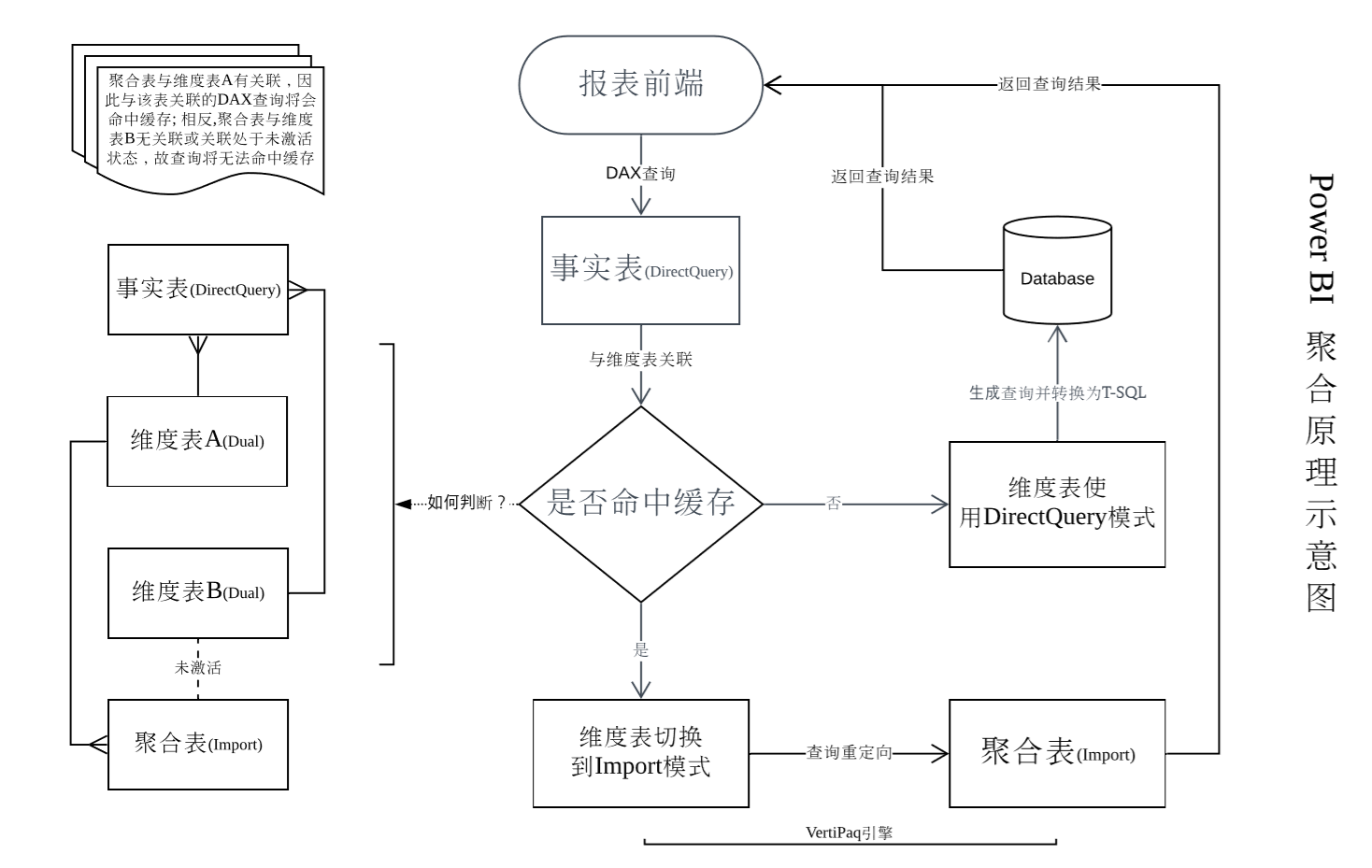### 实践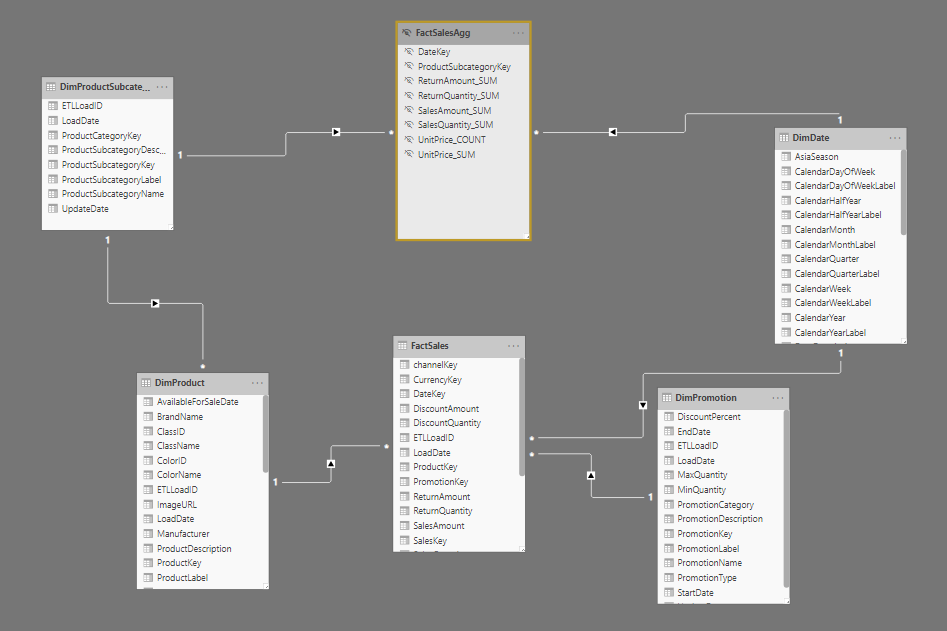``````SELECT [DateKey]
,[ProductSubcategoryKey]
,SUM(P1.[UnitPrice]) AS [UnitPrice_SUM]
,COUNT(P1.[UnitPrice]) AS [UnitPrice_COUNT]
,SUM([SalesQuantity]) AS [SalesQuantity_SUM]
,SUM([ReturnQuantity]) AS [ReturnQuantity_SUM]
,SUM([ReturnAmount]) AS [ReturnAmount_SUM]
,SUM([SalesAmount]) AS [SalesAmount_SUM]
FROM [ContosoRetailDW].[dbo].[FactSales] P1
LEFT JOIN [ContosoRetailDW].[dbo].[DimProduct] P2
ON P1.ProductKey = P2.ProductKey
GROUP BY
[DateKey]
,[ProductSubcategoryKey]
``````

``````SalesAMT = SUM(FactSales[SalesAmount])
------------------------------------
SalesQty = SUM(FactSales[SalesQuantity])
``````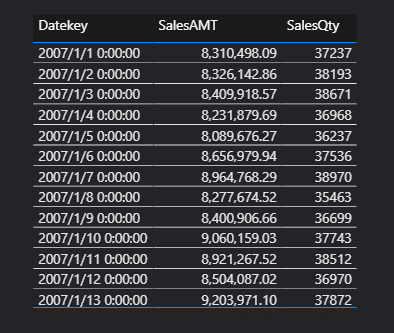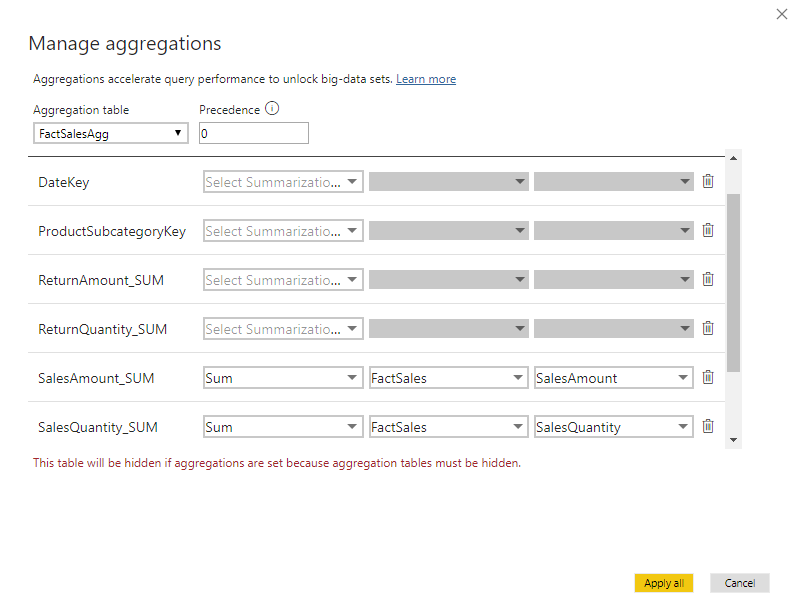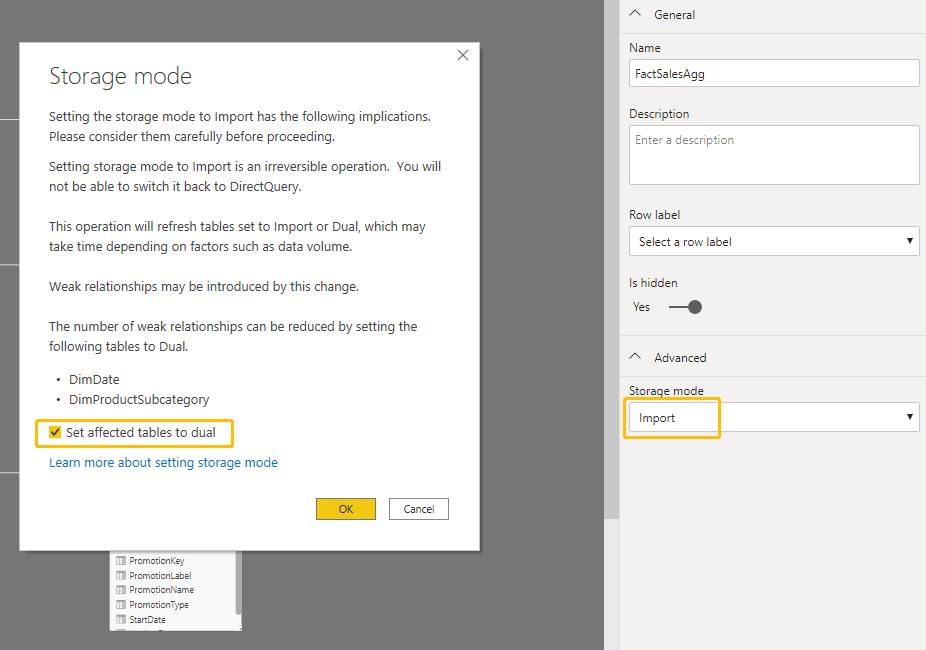### 验证